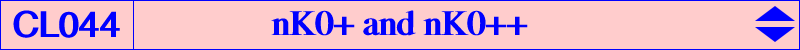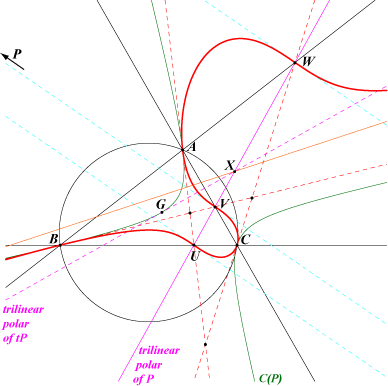Let us consider the nK0 with pole W = p:q:r and root P = u:v:w. This has equation ∑ux(ry^2+qz^2) = 0. It is the locus of point M such that M and its isoconjugate M* are conjugated in the circumconic with perspector P. This cubic is a nK0+ (i.e. has three - real or not - concurring asymptotes) if and only if its pole W lies on the cubic Kpole = nK(W0, G, P) whose pole is W0 = u^2(v + w - 2u) : : . Its equation is : (x + y + z) [∑ u^2(v + w - 2u)yz] = 2(u + v + w)[∑(u^2 - vw)] xyz (1) Kpole is a nK0 if and only if P lies on K015, the Tucker nodal cubic, in which case it is a nK0+.nK0+ with root G Since W0 is not defined for P = G, we must consider this special case separately. In fact, any nK0(W, G) is a nK0+ with three real asymptotes parallel to the sidelines of ABC and concurrent at W. The tangents at A, B, C are the sidelines of the anticevian triangle of W. The most interesting examples are : K016 = nK0+(X2, X2), the Tucker+ cubic, a member of the class CL026, K082 = nK0+(X6, X2), the equal power+ cubic, a member of the class CL020, K196 = nK0+(X76, X2), the isotomic transform of K024, the Kjp cubic.nK0+ with root at infinity We now suppose that P is not G. This gives ∑(u^2 - vw) ≠ 0. The equation (1) above shows that Kpole degenerates when P lies at infinity. It rewrites under the form (x + y + z) [∑ u^3 yz] = 0 showing that W must lie at infinity or on the circumconic (C3) with perspector P^3, the barycentric cube of P. P^3 is the intersection of : the tangent at P to the circum-conic through P and its isotomic conjugate tP, the tangent at tP to the conic through P, tP and the vertices of the anticevian triangle of P. 1. any nK0 with pole W and root P both at infinity is always a nK0+ with asymptotes concurring at G. It becomes a nK0++ (i.e. its asymptotes concur at G on the cubic) if and only if the directions given by W and P are conjugated in the Steiner ellipse. In such case, we obtain a central cubic of the class CL012. See K068, K398, K399, K400a and K400b for example. 2. any nK0 with root P at infinity and pole W on (C3) is a nK0+ with asymptotes concurring at X lying on another nodal circum-cubic with node G, passing through TG(P). (C3) contains a large number of reasonnably simple points which are the isoconjugates of the points lying on the polar line of P in the Steiner ellipse under the isoconjugation with fixed point P. Each point M on this line gives a point W on (C3) such that nK0(W, P) is a nK0+.For example, with M = G, we have W = P^2 and the nK0+ is nK0+(P^2, P). The (real or not) asymptotes concur at the intersection X of the trilinear polars of P and tP. Since P = P*, the tangents at the third points U, V, W on the sidelines of ABC are parallel and the polar conic of P is the line UVW (the trilinear polar of P) counted twice. It follows that U, V, W are the real inflexion points on the cubic. The hessian degenerate into three lines : one is the line UVW and the two other are imaginary and parallel with infinite point P.Properties of Kpole and other nK0+ We now suppose that P is a finite point distinct of G and let TG(P) be the tripolar centroid of P. TG(P) is the isobarycentre of the traces of the trilinear polar T(P) of P on the sidelines of ABC. TG(P) has coordinates u(v-w)(v + w - 2u) : : . Recall that Kpole is nK(W0, G, P) with W0 = u^2(v + w - 2u) : : , obviously on the line through P^2 and P^3. Apart A, B, C, the infinite points of the sidelines of ABC, the cubic Kpole contains the following points, each being the pole of a (possibly decomposed) nK0+ with root P : • P with a tangent parallel to the trilinear polar of tP. This gives the cubics nK0+(P, P) of the class CL026. This type of cubic has a pencil of circular polar conics if and only if P lies on the orthic axis. • P* = u(v + w - 2u) : : , the W0-isoconjugate of P, a point on T(P). The cubics nK0+(P*, P) have their asymptotes concurring at TG(P). They are nK0++ if and only if P lies on K015. nK0+(P*, P) has a pencil of circular polar conics (as in Table 47) if and only if P lies on the Thomson cubic. See K393 and K604 for example and also the related quintic Q087. • P1 = u^2[v^2 + w^2 - u(v + w)] / (v - w) : : , the third point on PP*. • P1* = (v - w)(v + w - 2u) / [v^2 + w^2 - u(v + w)] : : . P1* is the common tangential of P and P*. • P2 = 1 / (v - w) : : , the trilinear pole of the line GP, on the Steiner ellipse. • P2* = u^2(v - w)(v + w - 2u) : : , but nK0+(P2*, P) decomposes into T(P) and the circum-conic with perspector TG(P). • P3 = PP2 /\ P*P2*. • P3* = PP2* /\ P*P2. • P4 = (v-w)(v + w - 2u) / (v+w-u) : : , on the lines P1P2 and P1*P2*, giving nK0++(P4, P) which is a central cubic with center (v + w - 2u) / (v + w - u) : : . • P4* = u^2(v + w - u) / (v - w) : : , on the lines P1*P2 and P1P2*. • P5 = P3P4 /\ P3*P4*. • P5* = P3P4* /\ P3*P4.Special nK0+ A nK0+ is an equilateral cubic if and only if its pole lies on K396 and its root on K397. A nK0+ is circular if and only if its pole lies at infinity (in which case it decomposes) or on a complicated septic containing X(1576) (giving a decomposed cubic again), X(1989) giving K064, X(6) which is a triple point, giving three focal cubics with root on the orthic axis, singular focus on the circumcircle and on the Grebe cubic. These cubics belong to the pencil generated by the Brocard cubics K018, K019. They are members of the class CL025.